# ML Aggarwal Class 7 Solutions for ICSE Maths Chapter 5 Sets Objective Type Questions

## ML Aggarwal Class 7 Solutions for ICSE Maths Chapter 5 Sets Objective Type Questions

Mental Maths

Question 1.
Fill in the blanks:
(i) If x is not a member of the set A, then symbolically we write it as ……..
(ii) Each element of a set is listed once and only ……….. repetitions are removed.
(iii) A set that contains a limited number of different elements is called a ……… set.
(iv) Two finite sets are called equivalent if and only if they have ………. a number of elements.
Solution: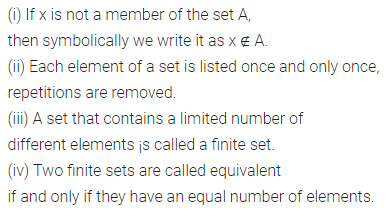Question 2.
State whether the following statements are true (T) or false (F):
(i) A collection of books is a set.
(ii) If X = {letters of the word PRINCIPAL}, then the cardinal number of set X is 9.
(iii) If P = {letters of the word AHMEDABAD}, then n(P) = 6.
(iv) If A is any set, then A ⊂ A.
(v) An empty set is a subset of every set.
(vi) If set A = {0}, then n{A} = 0.
(vii) If A and B are two sets such that A ⇔ B, then A = B.
Solution: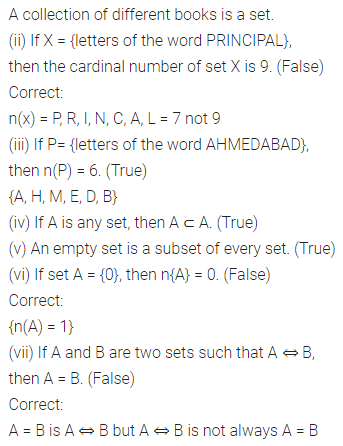Multiple Choice Questions

Choose the correct answer from the given four options (3 to 9):

Question 3.
Which of the following collection forms a set?
(a) Collection of 5 odd prime numbers
(b) Collection of 3 most intelligent students of your class
(c) Collection of 4 vowels of the English alphabet
(d) Collection of first 6 months of a year.
Solution: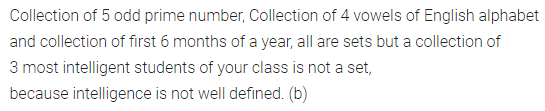Question 4.
The tabular form for the statement ‘Days of the week starting with the letter T’ will be
(a) {Days of week starting with letter T}
(b) {Tuesday, Thursday}
(c) {x | x is a day of the week starting with letter T}
(d) none of these
Solution:Question 5.
A set with a limited number of distinct elements is called
(a) a finite set
(b) an infinite set
(c) both finite as well as an infinite set
(d) none of these
Solution:Question 6.
The symbol ↔ stands for
(a) belongs to
(b) is a subset of
(c) is equivalent to
(d) none of these
Solution: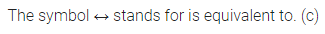Question 7.
The empty set is denoted as
(a) {Φ}
(b) { }
(c) {0}
(d) 0
Solution: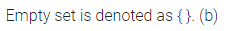Question 8.
The cardinal number n(A) = for A = {x : x is an odd prime number less than 20} is
(a) 8
(b) 7
(c) 9
(d) 10
Solution:Question 9.
If A = {x | x is a positive mutliple of 3 less than 20} and B = {x | x is a prime number less than 20}, then n(A) + n(B) is
(a) 6
(b) 8
(c) 13
(d) 14
Solution: